Skip to main content

# Energy-efficient downlink resource management in self-organized OFDMA-based two-tier femtocell networks

## Abstract

Femtocell is a novel technology that is used for escalating indoor coverage as well as the capacity of traditional cellular networks. However, interference is the limiting factor for performance improvement due to co-channel deployment between macrocells and femtocells. The traditional network planning is not feasible because of the random deployment of femtocells. Therefore, self-organization approaches are the key to having successful deployment of femtocells. This study presents the joint resource block (RB) and power allocation task for the two-tier femtocell network in a self-organizing manner, with the concern to minimizing the impact of interference and maximizing the energy efficiency. In this study, we analyze the performance of the system in terms of the energy efficiency, which is composed of both the transmission and circuit power. Most of the previous studies investigate the performance regarding the throughput requirement of the two-tier femtocell network while the energy efficiency aspect is largely ignored. Here, the joint allocation task is modeled as a non-cooperative game which is demonstrated to exhibit pure and unique Nash equilibrium. In order to reduce the complexity of the proposed non-cooperative game, the joint RB and power allocation task is divided into two subproblems: an RB allocation and a particle swarm optimization-based power allocation. The analysis of the proposed game is carried out in terms of not only energy efficiency but also throughput. With practical 3rd Generation Partnership Project (3GPP) Long-Term Evolution (LTE) parameters, the simulation results illustrate the superior performance of the proposed game as compared to the traditional methods. Also, the comparison is carried out with the joint allocation scheme which only considers the throughput as the objective function. The results illustrate that significant performance improvement is achieved in terms of energy efficiency with slight loss in the throughput. The analysis in regard to energy efficiency and throughput of the two-tier femtocell network is carried out in terms of the performance metrics, which include convergence, impact of varying RBs, impact of femtocell density, and the fairness index.

## Introduction

Femtocell is a promising technology for expanding indoor coverage as well as the capacity of traditional cellular networks . Therefore, it has attracted attention from academia, industry, and standardization forums. Although femtocell can escalate the performance of indoor users, interference is the critical factor in this regard because of the co-channel deployment of macrocell and femtocell. Femtocell is serviced by a small cellular base station (SBS), which is specifically designed for indoor users or small business. An important characteristic of femtocell is that they are installed randomly and connected to the core macrocell network by using an existing backhaul link, which could be digital subscriber line (DSL), optical fiber, etc. This backhaul link makes it possible for communication with the macrocells and femtocells. The benefits obtained by exploiting femtocell can be divided into two categories: operator and customer perspectives. From the operator’s perspective, femtocell alleviates the burden on the macrocell network by offloading the traffic from the macrocell to the femtocell network. As far as the customer is concerned, high throughput, reduced power, and reliable communication are achievable by the reduced distance between the femto users (FUEs) and the connected SBS .

A hierarchical two-tier femtocell network is usually implemented in a co-channel environment owing to the efficient spectrum utilization. The interference in this two-tier network is bottle neck in the overall performance. Principally, there are two types of interferences that exist in the two-tier femtocell network: cross-tier and co-tier . Within the context of femtocells, the former is the interference between macrocells and femtocells while the latter is among femtocells. Thereby, it is imperative and challenging to deal with the interferences in such an unplanned femtocell network.

Traditional network planning and interference management strategies are not feasible for the two-tier femtocell networks. This is in accordance to the fact that prior information regarding the positions and number of femtocells is generally unknown to the operators. Therefore, self-organization provides a vital solution for the management of this unplanned femtocell network. On the other hand, the interference can also be adequately managed by employing the self-organization approaches and consequently improves the system performance . The self-organization concept originates from cognitive radio technology in which there is a little or no involvement of centralized entity. Most of the existing literature on the two-tier network only considers the throughput as the performance measure of the network, while the energy efficiency aspect is largely ignored . For the dense deployment of femtocells, energy efficiency becomes a prime concern that needs significant attention. Besides this, the energy efficiency has recently attracted the attention from academia, industry, and standardization forums because of the rapid growth in the demand of users.

This motivates us to investigate the energy efficiency aspect for the self-organized two-tier femtocell networks.

### Contributions

In this study, the joint allocation task for the downlink two-tier femtocell networks is modeled as a non-cooperative game. Our main contributions in this study are categorically defined as under:

1. 1.

An energy-efficient resource block (RB) and power allocation task is modeled as a non-cooperative game for the two-tier femtocell network. Precisely, the players (SBSs) in the game interact with the environment autonomously and learn the action profile (RB and power levels) with the concern to maximizing the energy efficiency. Under the assumption of the availability of channel gains, the proposed game demonstrated to exhibit pure and unique Nash equilibrium.

2. 2.

The utility function of the proposed game is designed in a manner that maximizes the energy efficiency and minimizes the impact of interference in the two-tier network.

3. 3.

The complexity of the joint RB and power allocation task of the proposed game is decomposed into two subproblems: an RB allocation and a particle swarm optimization (PSO)-based power allocation. This decomposition significantly minimizes the complexity in each iteration of the proposed game.

4. 4.

The analysis of the proposed game is carried out regarding energy efficiency and throughput. Additionally, two different scenarios are taken into consideration for having an in-depth analysis of the proposed game. The comparison of the proposed game is carried out regarding joint allocation game with throughput maximization  and traditional methods.

5. 5.

Simulation results are computed regarding energy efficiency and throughput in terms of the performance measures which include convergence, impact of varying RBs, impact of femtocell density, and the fairness index.

### Related work

The related work is divided into three parts: different approaches for the self-organized resource management [6-10], the game-theoretic approaches for resource management [11-13], and the energy-efficient resource management for two-tier femtocell networks [14,15].

The authors in  propose a joint resource and power allocation in self-organized femtocell networks by exploiting a potential game. However, the whole analysis is carried out in terms of the throughput of the macrocell and femtocell networks. The authors in  propose a utility-based signal to interference and noise ratio (SINR) that reduces the cross-tier interference in a femtocell network. However, they do not cater the co-tier interference component, which is also termed as the bottle neck in performance enhancement for the shared channel environment. The authors in  propose a heuretic approach for resource allocation and power control for femtocell networks. However, the assumption of their study is that the information is thoroughly exchanged among the SBSs for performance improvement which is not practical in the real environment. The authors in  employ a novel docitive Q-learning for self-organized resource allocation in femtocell networks. However, it takes time to learn the learning mechanism for the optimal strategies, which makes it unsuitable for the real cases. The authors in  propose a joint subchannel and power allocation for the downlink of femtocell networks. Specifically, they have exploited the convex optimization and iterative approach for performance improvement of the network. However, they have only considered the throughput of the system and ignored the energy efficiency aspect that we are presenting in this study.

Game-theoretic approaches, which are appropriate for distributed resource management, have been extensively applied for the two-tier femtocell networks. The authors in  present a joint channel allocation and power control by using game learning mechanisms for cognitive radio networks. They utilize the regret learning method for convergence to the Nash equilibrium. Moreover, the comparison with genetic algorithm in centralized framework justifies their mechanism. The results illustrate that the inculcation of no-regret learning within the context of no-cooperation game theory has similar performance to that of the centralized one at the cost of increased complexity. The authors in  propose a distributed power allocation scheme using a game theoretic framework for the overlay scenario in cognitive radio networks. More precisely, the game theoretic proposition aims for maximizing the secondary users’ throughput while keeping the interference inculcated on other secondary users and primary networks below the specified threshold. However, the uplink transmission case consideration imposes a huge burden to the FUEs in the network. The authors in  present a novel Stackelberg game for the resource allocation problem within the context of femtocell networks. In the game, the macro base station (MBS) which is the leader selects the resources for meeting the demand of its users followed by the allocation of followers with the concern to maximizing the throughput. They take the spectrum sharing into account while assuming the fixed power. However, the joint allocating task significantly improves the performance. All the investigation [11-13] carried out only considers the throughput enhancement of the network without taking into consideration the energy efficiency.

Most of the literature focuses on the throughput enhancement of the network; however, the limited battery capacity leads to the attention on the energy efficiency aspect of the two-tier femtocell networks. Although the energy efficiency aspect is largely ignored, a few efforts have been made in this domain, which includes  and . The authors in  propose a non-cooperative game for subcarrier allocation and power control that aims at maximizing the energy efficiency. The authors in  investigate the non-cooperative power optimization game for enhancing energy efficiency. Both works  and  investigate the resource management for uplink case, which is indeed important for the limited power batteries. On the other hand, the growing demands of users and dense deployment of femtocells attract attention on the energy efficiency in the downlink of the femtocell networks. This will contribute to minimization of the energy consumption of the voice and data networks. Therefore, in this study, we are presenting the energy-efficient downlink resource management for the two-tier femtocell networks.

According to the best of the authors’ knowledge, the proposed game-based joint RB and power allocation of the two-tier femtocell networks is unique and has not been investigated so far. The exploitation of both RB and power allocation not only maximize the energy efficiency but also maximize the throughput which is shown in the results. This is in accordance to the fact that interference components are also included in the energy efficiency maximization expression which accordingly enhances the throughput also. On the other hand, the decomposition of the joint allocation task into RB allocation and PSO-based power allocation makes this study a unique one. The concern of the decomposition of the joint allocation task is to minimize the complexity which makes it more appropriate for real systems. On the other hand, two different scenarios are taken into account for having an in-depth analysis of the proposed game.

The rest of this paper is structured as follows: the system model and proposed framework are presented in Section 2. Energy-efficient non-cooperative game is presented in Section 3, which includes detailed information of the proposed game. The simulation results regarding the energy efficiency and throughput in terms of the performance measures are presented in Section 4. Finally, Section 5 concludes the article.

## System model

### Proposed framework

The considered framework for the proposed non-cooperative game for RB and power allocation in two-tier femtocell networks is shown in Figure 1, where macrocells are underlaid with multiple femtocells. Under the violation of the macro user (MUE) performance, MBS alters the concerning SBS to change the action profile accordingly. This information is conveyed by the existed backhaul link that connects MBS and SBS. The benefits gained from the proposed game are: First, energy efficiency is achieved for the two-tier femtocell network without jeopardizing the macrocell performance. Second, a less complex game is developed by decomposing the joint allocation task into two subproblems. Third, a self-organizing downlink resource management is achieved with no involvement of centralized entity.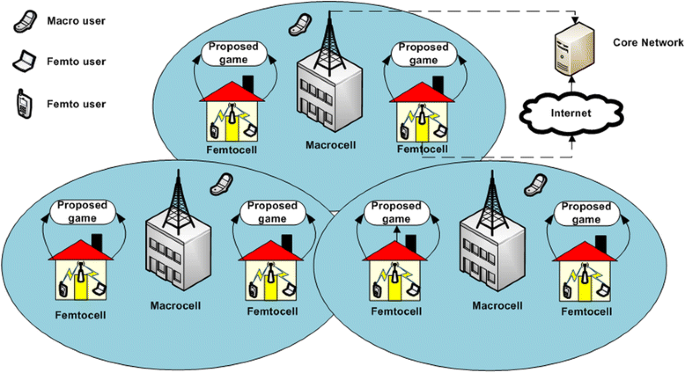Figure 1

The interference scenarios that we considered in this study are explicitly shown in Figure 2. Specifically, we are considering the downlink case of the two-tier femtocell networks. The cross-tier interference component which is the interference between the macrocell and femtocell is represented by i1. On the other hand, the co-tier interference which is the interference among femtocells is depicted by i2 and i3. Owing to the co-channel environment, the aforementioned interference creates significant impact on the performance of the network. The proposed non-cooperative game-based joint resource management mitigates both the interference components and enhances the energy efficiency accordingly.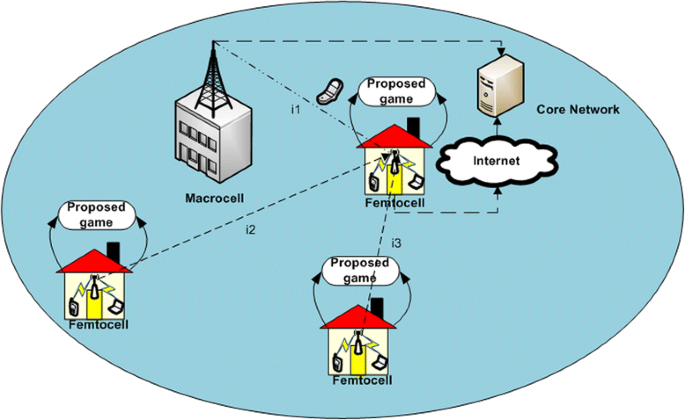Figure 2

### Energy efficiency performance criterion

The repeatedly growing demands of users attract the attention from academia, industry, and standardization forums towards the energy-efficient design of wireless networks [16-19]. Generally, there are two methods for computing the energy efficiency of the system. The first one considers the amount of information in bits transmitted per Joule (bits/J) [16,17], while the other takes into account the transmission power [18,19].

In this study, we are using the first energy-efficient performance criterion which is the ratio of the transmission capacity to the transmission power [16,17]. Moreover, the transmission power also contains the circuit power. The energy efficiency (EE) in bits/J for the OFDMA-based system can be written as:

$$\mathrm{E}\mathrm{E}=\frac{W{ \log}_2\left(1+\frac{h^2p}{\sigma^2}\right)}{p_c+p}$$
(1)

where W is the bandwidth, р c is the circuit power, р is the transmission power, h is the channel gain, and σ 2 is the noise power. The reason behind the exploitation of the energy efficiency performance metric is to transmit more information using less power.

### Problem formulation

In this study, we consider a two-tier OFDMA-based femtocell network comprising of L macrocells, where each macrocell is serviced by an MBS at its center. In addition, each macrocell is overlaid with N femtocells, where each femtocell is serviced by an SBS. Specifically, we are considering the downlink case for the resource management in the two-tier femtocell network along with the universal frequency reuse-1 for each cell. The concern of employing the frequency reuse-1 is due to the fact that each cell has access of all the pool of RBs. The total Y MUEs are randomly deployed within the coverage area of a macrocell, and X FUEs are assumed to be in the indoor environment. The closed group formation (CSG) within the femtocell is assumed in which a certain number of FUEs can only be a part of the femtocell network. Concerning the co-channel deployment, the total pool of Q RBs is taken into account here. On the other hand, the number of RBs acquired by each SBS at any time is assumed to be G such that GQ. In addition, the total R power levels are considered which can be utilized by each SBS on the acquired G RBs under the constraint of maximum power transmission. The tight synchronization amount the OFDMA subcarriers is taken into account, which correspond to the fact that interference is only inculcated when there is transmission on the same RBs.

The transmission power of lth MBS and nth FBS on acquired G RBs is given by $${p}_l^{g,M}=\left\{{p}_l^{1,M}, \dots, {p}_l^{G,M}\right\}$$ and $${p}_n^{g,F}=\left\{{p}_n^{1,F}, \dots, {p}_n^{G,F}\right\}$$. The maximum power constraint on each base station is represented as $${P}_{\mathrm{MAX}}^M$$ and $${P}_{\mathrm{MAX}}^F$$ such that $${\sum}_{g=1}^G}{p}_n^{g,M}\le {P}_{\mathrm{MAX}}^M$$ and $${\sum}_{g=1}^G}{p}_n^{g,F}\le {P}_{\mathrm{MAX}}^F$$, respectively.

The performance of the proposed non-cooperative game is then analyzed in terms of the energy efficiency and throughput. In the computation of SINR ratio for femtocells, we evaluate the average SINR with respect to users on each RB. To this end, the SINR at the xth FUE of nth SBS operating on th $$g$$th RB is given by,

$${\mathrm{SINR}}_n^{g,F}=\frac{1}{X}\sum_{x=1}^X\frac{p_n^{g,F}{h}_{nn,x}^{g,F-F}}{\sigma^2+\underset{\mathrm{Femtocomponents}}{\underbrace{\sum_{i=1,i\ne n}^N{p}_i^{g,F}{h}_{in,x}^{g,F-F}{\delta}_{k_g{k}_i}}}}+\underset{\mathrm{Macrocomponents}}{\underbrace{\sum_{l=1}^L{p}_l^{g,M}{h}_{\ln, x}^{g,M-F}{\delta}_{k_g{k}_l}}}}}},\begin{array}{cc}\hfill \hfill & \hfill \hfill \end{array}$$
$$\forall n=1,\dots, N,g=1,\dots, G,x=1,\dots, X$$
(2)

where $${h}_{nn,x}^{g,F-F}$$ is the channel gain between xth FUE and nth SBS operating on th $$g$$th RB; $${h}_{in,x}^{g,F-F}$$ is the channel gain between xth FUE of nth SBS and ith SBS operating on th $$g$$th RB; $${h}_{\ln, x}^{g,M-F}$$ is the link gain between xth FUE of nth SBS and lth MBS operating on th $$g$$th RB; $${\delta}_{k_g{k}_l}$$ is the interference function that corresponds to the inculcation of the interference for the same set of RBs, i.e., if k g = k l , then $${\delta}_{k_g{k}_l}$$ = 1, otherwise, $${\delta}_{k_g{k}_l}$$ = 0, and finally, σ 2 is the noise power. In addition, the femto components are the co-tier interference components, whereas the macro components are the cross-tier one.

The SINR at yth MUE of lth MBS that have allocated th $$g$$th RB is given by,

$${\mathrm{SINR}}_l^{g,M}=\frac{1}{Y}\sum_{y=1}^Y\frac{p_l^{g,M}{h}_{ll,y}^{g,M-M}}{\sigma^2+\underset{\mathrm{Macrocomponents}}{\underset{\operatorname{}}{\sum_{i=1,i\ne l}^L{p}_i^{g,M}{h}_{il,y}^{g,M-M}{\delta}_{k_g{k}_i}}}}+\underset{\mathrm{Femtocomponents}}{\underbrace{\sum_{n=1}^N{p}_n^{g,F}{h}_{nl,y}^{g,F-M}{\delta}_{k_g{k}_l}}}}}},\begin{array}{cc}\hfill \hfill & \hfill \hfill \end{array}$$
$$\forall l=1,\dots, L,g=1,\dots, G,y=1,\dots, Y$$
(3)

The femtocell capacity of the nth SBS that has acquired G RBs among the Q pool of RBs is given by,

$${\varDelta}_n^F=\frac{W}{G}\begin{array}{cc}\hfill \sum_{g=1}^G}{ \log}_2\left(1+{\mathrm{SINR}}_n^{g,F}\right),\hfill & \hfill \hfill \end{array}\forall n=1,\dots, N$$
(4)

The energy efficiency of the nth SBS is represented as:

$${\mathrm{EE}}_n^F=\frac{\frac{W}{G}{\sum}_{g=1}^G{ \log}_2\left(1+{\mathrm{SINR}}_n^{g,F}\right)}}{{\sum}_{g=1}^G\left({p}_n^{g,F}+{p}_c\right)}},\forall n=1,\dots, N$$
(5)

where p c is the circuit power and W is the total system bandwidth.

The total energy efficiency of the two-tier femtocell networks is given by,

$${\mathrm{EE}}^F=\sum_{n=1}^N{\mathrm{EE}}_n^F={\sum}_{n=1}^N\frac{{\sum}_{g=1}^G\frac{W}{G}{ \log}_2\left(1+{\mathrm{SINR}}_n^{g,F}\right)}}{{\sum}_{g=1}^G\left({p}_n^{g,F}+{p}_c\right)}}}}$$
(6)

Concerning the joint RB and power allocation, the optimization problem is formulated as:

$$\max \frac{\frac{W}{G}{\sum}_{g=1}^G{ \log}_2\left(1+{\mathrm{SINR}}_n^{g,F}\right)}}{{\sum}_{g=1}^G\left({p}_n^{g,F}+{p}_c\right)}},\forall n=1,\dots, N,G\in \left\{1,2,\dots, Q\right\}$$
(7)

s.t.

$$\mathrm{C}1:\;\sum_{g=1}^G}\frac{W}{G}{ \log}_2\left(1+{\mathrm{SINR}}_n^{g,F}\right)\ge {\varGamma}_F,\forall n$$
$$\mathrm{C}2:\;\sum_{g=1}^G}\frac{W}{G}{ \log}_2\left(1+{\mathrm{SINR}}_l^{g,M}\right)\ge {\varGamma}_M,\forall l$$
$$\mathrm{C}3:\sum_{g=1}^G}{p}_n^{g,F}\le {P}_{\mathrm{MAX}}^F,\forall n$$
$$\mathrm{C}4:{p}_n^{g,F}\in \left[0,\frac{P_{\mathrm{MAX}}^F}{\mathrm{G}}\right],\forall n,g$$
$$\mathrm{C}5:\frac{1}{X}\sum_{x=1}^X}\left(\sum_{g=1}^G}\sum_{l=1}^L}{p}_l^{g,M}{h}_{\ln, x}^{g,M-F}\right)\le {\zeta}_n^{M-F},\forall n,x$$
$$\mathrm{C}6:\frac{1}{X}\sum_{x=1}^X}\left(\sum_{g=1}^G}\sum_{i=1}^{N-1}}{p}_i^{g,F}{h}_{in,x}^{g,F-F}\right)\le {\zeta}_n^{F-F},\forall n,x$$

The optimization problem in (6) aims at maximizing the energy efficiency subject to the minimum throughput requirement of the femtocells C1 and the maximum power constraint C3. Additionally, per RB power constraint is also taken into account as in C4. The performance of the macrocell users is protected by the incorporation of the constraint C2 in the problem formulation. The accumulated cross-tier and co-tier interference components are represented by the constraints C5 and C6. The concern of inculcating the aforementioned interference limits is that both the energy efficiency and throughput are enhanced accordingly.

### Notations and assumptions

The notations presented in Table 1 are used in the rest of the paper.

## Energy-efficient non-cooperative game-based resource block and power allocation

In this section, the energy-efficient non-cooperative game is presented, including modeling of the game as RB and power allocation, existence to the pure and unique Nash equilibrium, proposed self-organizing framework, and algorithmic details. The game theoretic approaches are the most suitable for the resource management of the self-organized femtocell network. Specifically, non-cooperative game has been extensively applied for the resource allocation problems for the wireless networks .

### Non-cooperative game

We model the joint RB and power allocation problem as a non-cooperative game. Generally, the game is represented by a tuple $$\mathcal{G}=\left\{N,\;\left\{{A}_n\right\},{\left\{{U}_n(.)\right\}}_{n\epsilon N}\right\}$$, where N is the finite set of players, A n is the set of action or strategies (action profile) that each player can opt, and U n is the utility function that is associated with each player. In other words, the utility function corresponds to the level of satisfaction of each nth player. Moreover, the utility function depends not only on the action profile of nth player A n but also on the other ones A n = {A 1, A 2,…, A n − 1, A n + 1,…, A N }. Precisely, the utility function can be thought of as a function that maps the action profile A n into a real number such that U n : A n . In a game, the general goal of each nth is to maximize its utility function while keeping the interest of other players into account.

In the proposed game, the players are the set of SBSs which interacts with the environment in a self-organizing manner for deciding the best action profile. The action profile associated with each nth player is composed of two parts: RB and power levels. Mathematically, the action profile can be written as $${A}_n=\left({S}_n^F,{P}_n^F\right)$$, where $${S}_n^F=\left\{{S}_n^1,{S}_n^2,\dots, {S}_n^G\right\}$$ and $${P}_n^F:{\sum}_{g=1}^G}{p}_n^{g,F}\le {P}_{\mathrm{MAX}}^F$$. In other words, $${S}_n^F$$ is the selection of G RBs by each nth SBS, and $${P}_n^F$$ is the power value on the selected RBs. Each player involved in a game makes the decision in a self-organizing manner, and their decision has strong impact on others. The concern of the study is the maximization of the energy efficiency of the two-tier femtocell network without compromising the macrocell performance. Therefore, the utility function U n is the same as the energy efficiency and is written as:

$${U}_n\left({A}_n,{A}_{-n}\right)=\frac{\frac{W}{G}{\sum}_{g=1}^G{ \log}_2\left(1+{\mathrm{SINR}}_n^{g,F}\right)}}{{\sum}_{g=1}^G\left({p}_n^{g,F}+{p}_c\right)}},\forall n=1,\dots, N$$
(8)

Definition 1: Given the fixed $${S}_{-n}^F$$ and $${P}_{-n}^F$$, the best response dynamics of the RB and power allocation are given by,

$$\left({S}_n^F,{P}_n^F\right)= \arg \underset{S_n^F,{P}_n^F}{\ \max\ }{U}_n\left({S}_n^F,{P}_n^F\Big|{S}_{-n}^F,{P}_{-n}^F\right)$$
(9)

where $${S}_{-n}^F$$ and $${P}_{-n}^F$$ are the action profile of all the players except the nth one.

### Existence and uniqueness of Nash equilibrium

A set of action profile is termed as Nash equilibrium if the players involve in the game cannot deviate profitably given the action profile of other players . For the proposed game, the Nash equilibrium is defined as:

Definition 2: A set of resource blocks and power allocation action profile $${A}^{*}=\left\{{A}_1^{*},{A}_2^{*},\dots, {A}_N^{*}\right\}$$ is termed as the Nash equilibrium if and only the following condition is satisfied,

$${U}_n\left({A}_n^{*},{A}_{-n}^{*}\right)\ge {U}_n\left({A}_n,{A}_{-n}^{*}\right)\forall n=\left\{1,2,\dots N\right\}$$
(10)

where $${A}_{-n}^{*}=\left\{{A}_1^{*},{A}_2^{*},\dots, {A}_{n-1}^{*},{A}_{n+1}^{*}, \dots, {A}_N^{*}\right\}$$ is the composite action profile of all the players in the Nash equilibrium except the nth player. The composite action profile corresponds to the RB and power allocation.

Primarily, the Nash equilibrium of the proposed game exists if the following two conditions are satisfied :

1. 1)

The action profile A n is a non-empty, convex, and compact subset of some Euclidean space .

2. 2)

The utility function U n (A n , A n ) is a continuous and quasi-concave.

Since the transmission power and RBs are finite non-empty sets, therefore, condition 1 is satisfied for each femtocell. As far as condition 2 is concerned, the utility function in (8) is differentiable and strictly concave with respect to the composite action profile (RB and power levels) . Thus, condition 2 is also satisfied, and the existence of the Nash equilibrium is guaranteed in accordance to the aforementioned conditions. We have the following theorems.

• Theorem 1: The Nash equilibrium point exists in the proposed game $$\mathcal{G}$$.

• Theorem 2: The proposed game has a unique Nash equilibrium .

### Resource block and power allocation

In this subsection, we describe the RB and power allocation of the proposed game. Generally, the optimal joint RB and power allocation is an NP-hard problem . In this manner, to reduce the complexity of the proposed game, the joint allocation task is decomposed into two subproblems: an RB allocation and a PSO-based power allocation. Primarily, in each iteration of the proposed game, two steps need to be carried out: RB allocation and power allocation. Firstly, the RB allocation is done given the power allocation of all the players. Secondly, the power allocation on the selected RBs is executed out which have been selected in the previous step. In both steps, the goal is to maximize the energy efficiency under the network constraints C1 to C6. The detail of the individual step is elaborated as below.

#### Resource block allocation

In this subsection, we describe the RB allocation algorithm which is based on the algorithm in . However, the difference here is that we analyze the energy efficiency rather than the throughput. On the other hand, the multichannel environment is considered in our proposed game. Specifically, each player (SBS) in our proposed game acquires G RBs at any time among the total Q RBs. Under the assumption of the availability of link gains of all the other players, the individual RB problem can be written as:

$${S}_n^{*F}=\underset{S_n^F}{ \max\ }{U}_n\left({S}_n^F\Big|{P}_{-n}^F\right)$$
(11)

It can be noticed from the above expression that the concern of the RB allocation step is to maximize the utility function, which indirectly maximizes the energy efficiency. The assumption here is that power levels of the other players are acquired on each player, and this information is repeatedly acquired via control channels from the existed backhaul link.

Substituting Equations (2) and (5) in (11), we get,

$${S}_n^{*F}=\underset{S_n^F}{ \max}\frac{{\sum}_{g=1}^G\frac{W}{G}\left(1+\frac{p_n^{g,F}{h}_{nn}^{g,F-F}}{I_n^{g,F}}\right)}}{{\sum}_{g=1}^G\left({p}_n^{g,F}+{p}_c\right)}}$$
(12)

where $${I}_n^{g,F}={\sigma}^2+{\sum}_{i=1,i\ne n}^N}{p}_i^{g,F}{h}_{in}^{g,F-F}{\delta}_{k_g{k}_i}+{\sum}_{i=1}^L}{p}_l^{g,F}{h}_{ln}^{g,M-F}{\delta}_{k_g{k}_i}$$ is the interference comprising of cross-tier and co-tier components. Assuming that ρ is the SINR value and substituting the power values of the SBSs $${p}_n^{g,F}=\frac{\rho {I}_n^{g,F}}{h_{nn}^{g,F-F}}$$ in (12), we get

$${S}_n^{*F}=\underset{S_n^F}{ \max}\frac{{\sum}_{g=1}^G\frac{W}{G}\left(1+\rho \right)}}{{\sum}_{g=1}^G\left(\frac{\rho {I}_n^{g,F}}{h_{nn}^{g,F-F}}+{p}_c\right)}}$$
(13)

It can be intuitively seen that the above expression be written as:

$${S}_n^{*F}=\underset{S_n^F}{ \min }{\sum}_{g=1}^G}\frac{I_n^{g,F}}{h_{nn}^{g,F-F}}$$
(14)

The above expression reveals that the contiguous group of G RBs is allocated by each SBS whose accumulated $${\sum}_{g=1}^G}\frac{I_g^{g,F}}{h_{nn}^{g,F-F}}$$ value is minimum.

#### Power allocation

In the power allocation step, the PSO is exploited for obtaining the optimum power values on the selected RBs in the previous step. The reason for incorporating the PSO is that it is less complex and gives better results in less time. Owing to the various considered power levels, the PSO-based power allocation evaluates the best power values and minimizes the complexity of the game. On the other hand, the main concern of breaking the joint allocation task into two subproblems is to minimize the complexity of the proposed game.

#### Particle swarm optimization

PSO is a population-based biologically inspired algorithm, inspired by the bird flocking and fish schooling mechanisms. These types of algorithms are specifically useful where the sample space is very large, the parameters of interest are dynamic, and there is little information exchanged between the users (particles) .

Generally speaking, the PSO algorithm starts with populating the swarm of particles, where each particle represents a potential solution. The swarm is similar to the population, while a particle is equivalent to the individual. Each particle is associated with a position and velocity in search space. In each iteration of the algorithm, the fitness is computed using (8) and both the velocity and position of each particle are updated according to (15) and (16), respectively. For the PSO-based power allocation, the fitness function is the same as the utility function that we have designed for the proposed game. The velocity of each particle is updated according to the finest two known positions, the personal best position (pbest) and the neighborhood best position (nbest), where pbest is the best position that the individual particle has visited and nbest is the best position that the particle and its neighborhood have visited. When the whole swarm is considered as the neighbor, then the nbest is termed as global best gbest and for small neighborhood nbest is equivalent to local best lbest.

$${V}_j^{\mathrm{new}}=\begin{array}{cc}\hfill {V}_j+{a}_1{r}_1\left({\mathrm{pbest}}_j-{O}_j\right)+{a}_2{r}_2\left({\mathrm{nbest}}_j-{O}_j\right)\hfill & \hfill \hfill \end{array}j=1,2,\dots, T$$
(15)
$${O}_j^{\mathrm{new}}={O}_j+{V}_j^{\mathrm{new}}$$
(16)

where:

• a 1 and a 2 are termed as acceleration coefficients whose job is to control the influence in the search process.

• T is the number of particles in a swarm.

• r 1 and r 2 are two random numbers uniformly distributed in the interval from (0, 1).

• O j , $${O}_j^{\mathrm{new}}$$, V j , and $${V}_j^{\mathrm{new}}$$ represent the current and updated position and velocity of the jth particle.

#### Particle swarm optimization-based power allocation

In a PSO-based power allocation step, the power levels on the selected RBs are evaluated with the concern to maximizing the energy efficiency. Under the assumptions of the availability of the power values of the other N – 1 players, the power allocation problem can be written as:

$${P}_n^{*F}=\underset{P_n^F}{ \max\ }{U}_n\left({P}_n^F\Big|{P}_{-n}^F\right)$$
(17)

The abovementioned power allocation is achieved by exploiting the PSO-based optimization. Principally, there are three main steps in the PSO-based optimization: particle encoding, fitness computation, and velocity and position upgradation. In our considered PSO-based power allocation, each particle is represented by a G-dimensional vector, where each element represents the power level utilized by the SBS. In addition, R power levels can be exploited here with the concern to maximizing the utility function. As far as the fitness of the particle is considered, we use the utility function in (8) as the fitness function for the PSO-based power allocation. More precisely, the PSO iteratively maximizes the utility function and yields best results in terms of power allocation on the selected group of G RBs by each SBS. The velocity and position of particles is upgraded by (15) and (16).

### Proposed self-organizing framework and algorithm

The proposed self-organizing framework is shown in Figure 3. Specifically, the framework comprises of three main phases: sensing, learning, and tuning. These three phases actually resemble the cognitive radio cycle . This self-organizing procedure is executed by each SBS involved in a game.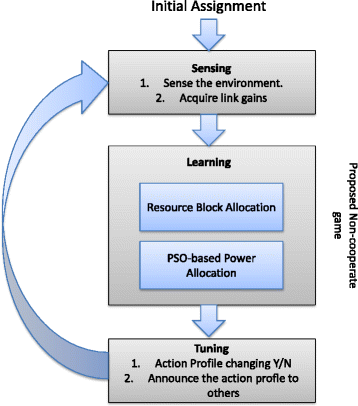Figure 3

Initially, the random assignment is done in terms of RBs and power levels by each SBS. In the sensing phase, each SBS interacts with the environment and acquires link gains. Here, we assume that the availability of link gains is strictly known on the SBSs, and this leads to the optimal performance of the proposed game in terms of the Nash equilibrium. In the learning phase, the proposed game is executed by each SBS based on the availability of link gains which are acquired in sensing phase. Concerning the high complexity of the joint RB and power allocation task, the joint problem is decomposed into two main tasks: an RB allocation and a PSO-based power allocation. In the RB allocation step, each SBS acquires the required group of G RBs according to (14) under the available of power levels of other players. In the second step, the power allocation is carried out on the selected RBs by exploiting PSO. Because of the various choices of power levels, the PSO-based optimization rules out the power levels in less time with the concern to maximizing the energy efficiency. Lastly, in the tuning phase, the decision is to be made by each SBS, i.e., whether to change the action profile or not. In addition, the changed action profile is also broadcasted to the neighbors so that they can also tune themselves accordingly.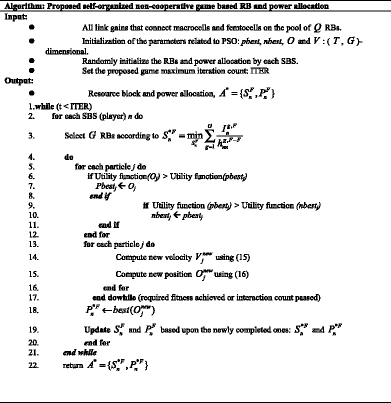As far as the complexity of the proposed non-cooperative game is concerned, the joint allocation task that we have considered in the game is decomposed into two subproblems: an RB allocation and a PSO-based power allocation. In each iteration of the proposed game, RB allocation and power allocation are carried out separately, and this significantly reduces the complexity of the original joint allocation task. Firstly, RB allocation is carried out, and then the PSO-based power allocation executes on the selected RBs which decides the optimal power levels on them. The concern of exploiting PSO for power allocation is that it gives the optimal power values without parsing the whole set of power values. Under the assumption of the availability of channel gains on the SBS, the proposed non-cooperative game converges to pure and unique Nash equilibrium. This information about the channel gains is repeatedly acquired on the control channels and helps in achieving the best performance. The proposed game is also scalable in the sense that any SBS can be in the part of the game any time. However, this may lead to a slightly slower convergence to the Nash equilibrium, where the convergence corresponds to the energy efficiency maximization of the femtocell networks without compromising the macrocell performance. As far as the comparison with the joint scheme is concerned, the joint optimization task is computationally intense.

## Simulation results and analysis

In this section, the simulation setup of the considered femtocell environment is presented and then the results are presented thereafter. The analysis of the proposed game is carried out regarding energy efficiency and throughput of the femtocell networks. In addition, the performance is evaluated in terms of the performance measures such as convergence, impact of varying RBs, impact of femtocell density, and the fairness index. For the sake of comparison, the following methods have been taken into account: joint resource and power allocation game with throughput maximization (JRPAG-TM) , proportional fair-non-cooperative power optimization game (PF-NPOG), and proportional fair-fixed power (PF-FP). In JRPAG-TM, a potential game is utilized for enhancing the throughput without considering the energy efficiency. The concern of comparing the proposed game with JRPAG-TM is to classify the gains and losses in energy efficiency and throughput. Furthermore, the two power levels for PF-FP are incorporated: 10 and 17 dBm. In order to have an in-depth analysis, two scenarios are taken into consideration: scenario 1 and scenario 2. In scenario 1, the number of SBS is taken to be N = 20, while in scenario 2 N = 40. All the simulations of the proposed game are done in MATLAB.

### Simulation setup

We consider an OFDMA-based urban setting environment, operating at 1,850 MHz. For the sake of simplicity, we consider a singular macrocell environment L = 1 with a radius of 1,000 m. However, for multicell environment, the cross-tier interference components also become a critical factor along with the co-tier for single cell. The performance of the system degrades somehow because of the addition of this interference component. The achieved convergence for multicell environment is also reduced due to cross-tier interference component. In addition, there are N femtocells underlaid in a macrocell, where each femtocell is of radius 40 m. The Y MUEs and X FUEs are randomly deployed within the coverage area of macrocell and femtocell. An important clarification is to be made here is that we are considering the average SINR computation among the X FUEs and is in accordance to (2).

In our proposed game, each SBS operates in a self-organizing manner and rules out the action profile (RB and power levels) with the concern to maximizing the energy efficiency without compromising the macrocell performance. According to the 3rd Generation Partnership Project (3GPP) Long-Term Evolution (LTE) specifications, the total system bandwidth W = 1,850 MHz is taken into consideration, which is composed of several RBs. These RBs are utilized by both macrocells and femtocells because of the co-channel deployment. Quantitatively, the total pool of RBs Q = 50 is taken into account. Furthermore, the number of RBs that SBSs can acquire any time is assumed to be G = 5. In order to have a thorough analysis, various values of G are employed such as G = {2, 4, 6, 8, and 10}. As far as the power levels are concerned, we are incorporating R = 100 power levels uniformly distributed in the range from −80 to 23 dBm. The maximum power constraint that each SBS can utilize is $${P}_{\mathrm{MAX}}^F=23$$ dBm. Furthermore, CSG mode of operation of femtocell is concerned; in which only particular users can be part of the femtocell network. The static circuit power of p c = 100 mW is taken into account here for the computation of energy efficiency for the femtocell network.

The pathloss (PL) models that we have utilized in this study for the two-tier femtocell networks are in accordance to the 3GPP . The PL models are:

1. a)

From MBS to MUE or FUE (indoor): PL(dB) = 15.3 + 37.6 log10 d.

2. b)

From MBS to MUE or FUE (outdoor): PL(dB) = 15.3 + 37.6 log10 d.

3. c)

From SBS to MUE or FUE (different): PL(dB) = 7 + 56 log10 d + γ

4. d)

From SBS to FUE (own): PL(dB) = 37 + 20 log10 d + γ

where d is the distance between MBS/SBS and MUE/FUE, γ is the penetration loss and its value is taken to be 15 dB. The shadowing factor of 8 and 4 dB is also contemplated here for indoor and outdoor, respectively.

The proposed game is executed for 100 iterations, and within each iteration, each SBS interacts in a self-organizing manner for evaluating the resources until the Nash equilibrium is achieved. Additionally, the results are captured for 1,000 different independent instances of the game. In the PSO-based power allocation, the PSO is executed for 200 iterations for obtaining the optimum results in terms of maximize energy efficiency (fitness function). The parameters for the PSO utilized for the power allocation are given in Table 2.

### Simulations results

#### Convergence in terms of energy efficiency and throughput

The convergence characteristics of the proposed game in terms of energy efficiency and throughput for both the scenarios are depicted in Figures 4 and 5. It can be seen that energy efficiency of the proposed game for both the scenarios increases with the increase of game iterations as shown in Figure 4a,b. This is in accordance to the fact that each SBS competes for resources (RB and power levels) in a competitive manner until the optimal performance, i.e., the Nash equilibrium point is achieved. However, the proposed game in scenario 2 takes longer time to converge to the Nash equilibrium point. The reason is that the large number of SBSs (players) in scenario 2 leads to slower convergence to the Nash equilibrium because of increased competition. On the other hand, the addition of players in scenario 2 corresponds to the reduced energy efficiency. The comparison of the proposed non-cooperative game with JRPAG-TM, PF-NPOG, PF-FP (10 dBm), and PF-FP (17 dBm) illustrates the superior performance in both the scenarios. On the other hand, the comparison with PF-NPOG illustrates the significance of employing the joint allocation task within the game. In PF-NPOG, power is optimized in accordance with the utility function, while RBs are allocated randomly. Another important point to be noticed is that the performance gap between the proposed game and PF-NPOG becomes wider by increasing from N = 20 to 40 (scenario 1 to scenario 2). This is due to the random allocation of RBs, which degrades the performance with the increase of SBSs. The comparison with PF-FP with two different fixed power levels (10 and 17 dBm) also illustrates the superior performance of the proposed game. The concern of employing the energy efficiency within this framework is elaborated here, i.e., each scenario, to which a large power corresponds, reduces energy efficiency, and this is in accordance with the designed utility function for the proposed game.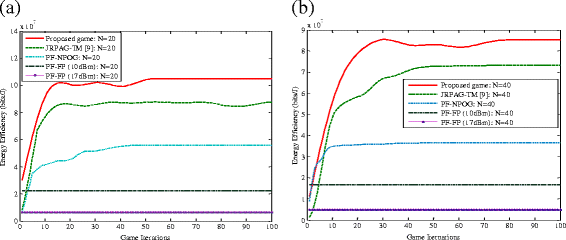Figure 4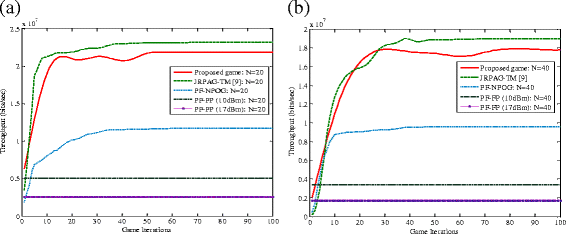Figure 5

Figure 5 illustrates the convergence in terms of throughput versus the game iterations for both the scenarios. The convergence in terms of throughput of the proposed game also increases with the increase of game iterations. Although the utility function of the game is designed by taking into account the energy efficiency, the throughput will also be adequately improved by taking into account various RBs and power levels. Similar reason for slow convergence holds for throughput in Figure 5 as in energy efficiency in Figure 4. The comparison with the PF-NPOG, PF-FP (10 dBm), and PF-FP (17 dBm) illustrates the superior performance of the proposed game in terms of throughput. However, the comparison with JRPAG-TM illustrates a slight loss in the throughput for each scenario. The reason being that in JRPAG-TM, the objective is solely to enhance the throughput without considering energy efficiency. In PF-NPOG, for each scenario, only power allocation is considered in the utility function of the game whereas the RBs are randomly allocated, and this corresponds to a significant inferior performance as compared to the proposed game. A similar performance trend with PF-FP exists as in energy efficiency. It can be seen that a larger fixed power of 17 dBm corresponds to reduced throughput, and this is due to the fact that utility function is designed in a manner that is aligned with the energy efficiency. Therefore, a better performance is achieved for reduced power in both the scenarios.

#### Impact of varying RBs on energy efficiency and throughput

The impact of varying RBs on energy efficiency and throughput of our proposed game is illustrated in Figures 6 and 7. The energy efficiency is plotted versus the number of RBs acquired by each SBS G in Figure 6. In addition, two different pools of resources are taken into consideration: Q = 25 and 50. It is illustrated in Figure 6a,b that energy efficiency of the proposed game for both the scenarios increases with the increase of G. The reason is that the increased number of RBs acquired per SBS results in the accumulation of energy efficiency values, and this is in accordance with (4). On the other hand, increase in the pool of RBs Q 25 to 50 also contributes to the escalation of energy efficiency for both the scenarios. However, the reduced energy efficiency value for scenario 2 is observed, and this is due to the increased number of SBSs. As far as the comparison of the proposed game with JRPAG-TM, PF-NPOG, PF-FP (10 dBm), and PF-FP (17 dBm) is concerned, the proposed game outperforms the considered methods. A performance gap of around 15% is observed while comparison with JRPAG-TM for each scenario. The comparison with the PF-NPOG illustrates that the performance gap is around 40% for scenario 1 while 50% for scenario 2. A high performance gap exists because in the proposed game, both the RB and power allocation is taken into considerations while in PF-NPOG RB allocation is done randomly. On the other hand, 10% increased performance improvement is observed for scenario 2, and this is due to the increased competition among players. The comparison with PF-FP for both the scenarios also illustrates a significant performance improvement. Precisely, PF-FP (10 dBm) exhibits higher energy efficiency value as compared to PF-FP (17 dBm). The reason is that the utility function is aligned with the energy efficiency, which contributes to the better performance with reduced power.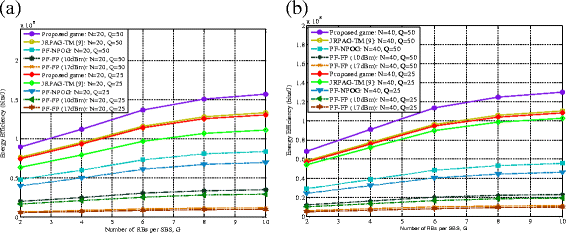Figure 6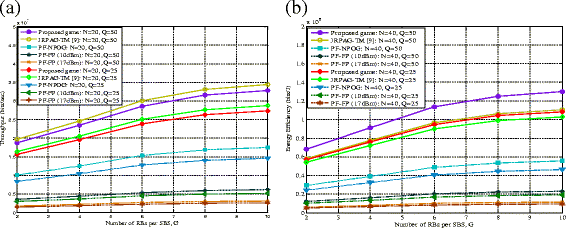Figure 7

Figure 7 illustrates the impact of varying the RBs on throughput of the femtocell network for both the scenarios. Firstly, the increasing trend of the proposed game in terms of throughput is observed by the increase of acquired RBs per SBS, i.e., G. Second, 20% increase in the throughput is observed by doubling the total pool of RBs from Q = 25 to 50. This is in accordance to the fact that accumulated throughput is achieved by the increase of G. On the other hand, the increase in the pool of RBs Q also contributes to the escalation of throughput for each scenario. Therefore, the throughput is enhanced with the increase of either G or Q for each scenario. Nevertheless, a reduced throughput for scenario 2 is also observed as compared to scenario 1, and this is due to the increase competition. The comparison of the proposed game with PF-NPOG, PF-FP (10 dBm), and PF-FP (17 dBm) in terms of throughput illustrates the superior performance of the proposed game.

Since the concern of the proposed game is to enhance the energy efficiency, a slight loss is observed while comparing with JRPAG-TM. However, the gain achieved in energy efficiency illustrates the supremacy of the proposed game as compared to JRPAG-TM. The throughput performance gap between proposed algorithm and PF-NPOG becomes wider with the increase acquired RBs per SBS. The reason is that in the proposed game, both the RB and power allocations are taken into account in the utility function, whereas in PF-NPOG, random allocation is employed which leads to reduced throughput. As far as the performance of the proposed game and PF-FP is concerned, a significant performance gap of around 490% in scenario 1 and 590% in scenario 2 is observed. The reason is that in PF-FP, both the RB and power levels are exploited without concerning the other players in the networks. Another important observation to be made here is that the reduced power levels in PF-FP (10 dBm) help in achieving high throughput as compared to PF-FP (17 dBm). This is due to the incorporation of the energy-efficient resource management which leads to better performance with reduced power levels.

#### Impact of varying femtocell cell density

The impact of femtocell density on energy efficiency and throughput is illustrated in Figure 8a,b. A significant performance trend gain about 15% is pertained while comparison of proposed game with JRPAG-TM. The energy efficiency of the proposed game decreases to about 90% with the increasing of femtocells from 10 to 80, whereas a sharp decaying effect is observed for PF-NPOG, i.e., about 250%. This is due to the fact that in the proposed game, both RB and power allocation are catered, whereas in PF-NPOG, only power allocation is exploited in utility function, and RBs are allocated randomly. On the other hand, a significant performance gap in terms of energy efficiency between the proposed game and PF-FP is also elaborated in Figure 8a. There is about 340% improvement with PF-FP (10 dBm) and 690% with PF-FP (17 dBm). In addition, this gap widens with the increase of femtocells.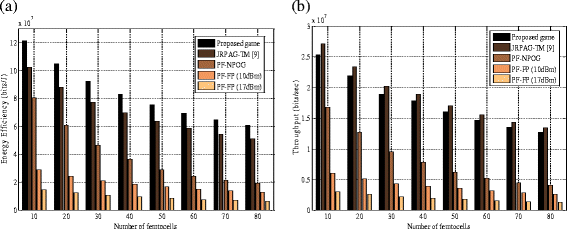Figure 8

The comparison in terms of throughput of the proposed game with traditional methods is illustrated in Figure 8b. The loss in the throughput of the proposed game as compared to JRPAG-TM is small of about 5%. The reason being that in JRPAG-TM, the concern is to maximize the throughput without considering energy efficiency. The throughput of the proposed game decreases gradually to about 90% while a sharp decaying effect is observed for PF-NPOG. However, an important investigation to be made here is that an PF-FP (10 dBm) has high throughput as compared to PF-FP (17 dBM). This is by virtue of the incorporation of energy efficiency in the utility that contributed to high throughput with reduced power values.

#### Fairness index

Figure 9 illustrates the fairness index comparison of the proposed game with traditional methods. Fairness is computed using Jain’s formula  as given below,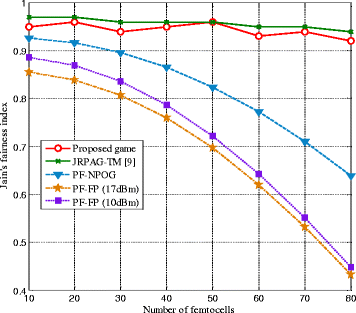Figure 9
$$f\left({n}_1,{n}_2,\dots, {n}_N\right)=\frac{{\left({\sum}_{i=1}^N{n}_i}\right)}^2}{N{\sum}_{i=1}^N{n}_i^2}}$$
(18)

where 0 ≤ f(n 1, n 2,…, n N ) ≤ 1, and this value corresponds to the satisfaction in terms of achieved average throughput. It can be seen that the fairness of the proposed game remains at a high level with the increase of femtocells. The fluctuations in the fairness curve of the propose game are due to the fact that the solution of the proposed game is suboptimal. And due to this, the satisfaction in terms of throughput of SBSs varies somehow which is shown in Figure 9. In other words, all the players involved in the game interact in a manner to obtain a stable Nash equilibrium point. The Nash equilibrium point is classified as a point at which none of the players can deviate profitably, and the requirements of all the players are satisfied in directly. However, the comparison with traditional methods reveals that superior performance of the proposed game exhibits in terms of the fairness index. As far as the comparison with the JRPAG-TM, there is a nominal increase in the fairness index values. However, the high energy efficiency gain illustrates the supremacy of the proposed non-cooperative game. On the other hand, the fairness value of the PF-NPOG drops to about 62% with the increase of femtocells from 10 to 80, and this is by virtue of the fact that RBs are allocated randomly in PF-NPOG. As far as the PF-FP with two power levels are concerned, their fitness values deteriorate progressively to about 43% because of the random and fixed power allocations. On the other hand, it can be seen that RA-FB (10 dBm) performs better as compared to RA-FB (17 dBm). The reason is that the exploitation of energy efficiency criterion leads to the increased energy efficiency and throughput for reduced power levels.

## Conclusions

In this study, an energy-efficient downlink resource management for two-tier femtocell networks is investigated. Specifically, the joint RB and power allocation are modeled here as a non-cooperative game in which the SBSs are the players and RB and power allocations are the action profiles. Most of the literature focuses only on the throughput enhancement of the two-tier system, while the energy efficiency aspect is largely ignored. To this end, the utility function of the proposed game is designed in a manner that is aligned with energy efficiency of the femtocell networks. The optimization problem is modeled as the maximization of the utility function (energy efficiency) subject to the network constraints C1 to C6. These network constraints not only satisfy the minimum throughput but also include the thresholds for interference components. Under the assumption of the availability of the link gains, the proposed game converges to pure and unique Nash equilibrium. Concerning the complexity of the joint allocation task, the problem is decomposed into two subproblems: an RB allocation and a PSO-based power allocation. The comparison of the proposed game with traditional methods illustrates the superior performance. In addition, the comparison with joint allocation task potential game, considering the throughput maximization, illustrates that significant performance in terms of energy efficiency with slight loss in throughput is achieved. The analysis is carried out in regard to energy efficiency and throughput.

## References

1. 1.

V Chandrasekhar, JG Andrews, A Gatherer, Femtocell networks: a survey. IEEE Commun Mag 46, 59–67 (2008)

2. 2.

FP7-ICT-2009-4, FREEDOM, Femtocell-based netwoRk Enhancement by intErference management and coorDination of infOrmation for seaMless connectivity, see official website: http://www.ict-freedom.eu/

3. 3.

N Saquib, E Hossain, BL Long, IK Dong, Interference management in OFDMA femtocell networks: issues and approaches. IEEE Wirel Commun 19, 86–95 (2012)

4. 4.

D Kinsely, T Yoshizawa, F Favichia, Standardization of femtocells in 3GPP. IEEE Commu Mag 47, 68–75 (2009)

5. 5.

Y Li, H Celebi, M Daneshmand, C Wang, W Zhao, Energy-efficient femtocells networks: challenges and opportunities. IEEE Wire Commun 20, 99–105 (2013)

6. 6.

A Shahid, S Aslam, HS Kim, K-G Lee, Distributed joint resource and power allocation in self-organized femtocell networks: a potential game approach. J Netw Comput Appl 46, 280–292 (2014). 7

7. 7.

V Chanrasekhar, JG Andrews, T Muharemovic, Z Shen, A Gatherer, Power control in two-tier femtocells networks. IEEE Trans Wirel Commun 8, 4316–4328 (2009)

8. 8.

A Shahid, S Aslam, KG Lee, Decentralized heuristic approach towards resource allocation in femtocells. Entropy 15, 2524–2547 (2013)

9. 9.

A Shahid, S Aslam, HS Kim, KG Lee, A docitive Q-learning for joint resource allocation and power control in self-organized femtocells networks. Trans. Emerging Tel. Tech. (2014) doi:10.1002/ett.2800

10. 10.

DT Ngo, S Khakurel, T Le-Ngoc, Joint sub channel assignment and power allocation for OFDMA femtocell networks. IEEE Trans Wire Commun 13, 342–355 (2014)

11. 11.

JR Gállego, M Canales, J Ortín, Distributed resource allocation in cognitive radio networks with a game learning approach to improve aggregate capacity. Ad Hoc Netw 10, 1076–1089 (2012)

12. 12.

T Nadkar, V Thumar, GPS Tej, SN Merchant, UB Desai, Distributed power allocation for secondary users in a cognitive radio scenario. IEEE Trans Wire Commun 11, 1576–1586 (2012)

13. 13.

P Xu, X Fang, M Chen, Y Xu, A Stackelberg game-based spectrum allocation scheme in macro/femtocell hierarchical networks. Computer Commun 36, 1552–1558 (2013)

14. 14.

S Buzzi, G Colavolpe, D Saturnino, A Zappone, Potential game for energy-efficient power control and subcarrier allocation in uplink multicell OFDMA systems. IEEE Sel Topics Sig Proc 6, 1923–4553 (2011)

15. 15.

GW Miao, N Himayat, GY Li, S Talwar, Distributed interference-aware energy-efficient power optimization. IEEE Trans Wire Commun 10, 1323–1333 (2011)

16. 16.

GW Miao, N Himayat, GY Li, D Bormann, in IEEE International Conference on Communications, 2008. ICC’08.Energy-efficient design in wireless OFDMA (Beijing, 19-23 May 2008), pp. 3307-3312

17. 17.

GW Miao, N Himayat, GY Li, Energy-efficient link adaptation in frequency-selective channels. IEEE Trans Commun 58, 545–554 (2010)

18. 18.

EA Jorswieck, H Boche, S Naik, Energy-aware utility regions: multiple access pareto boundary. IEEE Trans Wire Commun 9, 2216–2226 (2010)

19. 19.

Z Hasan, G Bansal, E Hossain, V Bhargava, Energy efficient power allocation in OFDMA-based cognitive radio systems: a risk-return model. IEEE Trans Wire Commun 8, 6078–6088 (2009)

20. 20.

K Akkarajitsakul, E Hossain, D Niyato, D Kim, Game theoretic approaches for multiple access network in wireless networks. IEEE Commun Surv Tutorials 13, 372–395 (2011)

21. 21.

D Fudenberg, J Tirole, Game theory (MIT Press, Cambridge, Massachussets, 1993)

22. 22.

J Zhang, T Lv, H Gao, Y Lu, in IEEE Wireless Communications and Networking Conference (WCNC), 2012. Joint uplink power and subchannel allocation in cognitive radio network (Paris, 1-4 April 2012), pp. 306–311

23. 23.

Z Chong-xian, L Chun-guo, Y Lu-xi, Dynamic resource allocation algorithm for multi-cell OFDMA systems based on noncooperative game theory. J Elect Infor Tech 8, 1935–1940 (2009)

24. 24.

H Izakian, A Abraham, V Snášel, Metaheuristic based scheduling meta-tasks in distributed heterogeneous computing systems. Sensors 9, 5339–5350 (2009)

25. 25.

J Mitola, Cognitive radio: an integrated architecture for software defined redio. PhD Thesis (Royal Institute of Technology (KTH), Sweden, 2000)

26. 26.

26. 3GPP TR 36.814, V.9.0.0 "Technical Specification Group Radio Access Network; Evolved Universal Terrestrial Radio Access (E-UTRA): Further advancements for E-UTRA physical layer aspects (Release 9)", March 2010

27. 27.

R Jain, D Chiu, W Hawae, A quantitative measure of fairness and discrimination of resource allocation in shared computer system, in DEC research report, 1984, p. TR-301

Download references

## Acknowledgements

This research was supported by the Ministry of Science, ICT and Future Planning (MSIP), Korea, under the Convergence Information Technology Research Center (Convergence-ITRC) support program (NIPA-2014-H0401-14-1006) supervised by the National IT Industry Promotion Agency (NIPA) and the Basic Science Research Program through the National Research Foundation (NRF) of Korea funded by the Ministry of Education, Science and Technology (2012009449).

## Author information

Authors

### Corresponding author

Correspondence to Kyung-Geun Lee.

## Additional information

### Competing interests

The authors declare that they have no competing interests.

## Rights and permissions

Open Access  This article is licensed under a Creative Commons Attribution 4.0 International License, which permits use, sharing, adaptation, distribution and reproduction in any medium or format, as long as you give appropriate credit to the original author(s) and the source, provide a link to the Creative Commons licence, and indicate if changes were made.

The images or other third party material in this article are included in the article’s Creative Commons licence, unless indicated otherwise in a credit line to the material. If material is not included in the article’s Creative Commons licence and your intended use is not permitted by statutory regulation or exceeds the permitted use, you will need to obtain permission directly from the copyright holder.

To view a copy of this licence, visit https://creativecommons.org/licenses/by/4.0/.

Reprints and Permissions